# NCERT Solutions for Class 9 Maths chapter 12 Exercise 12.1 - Heron’s Formula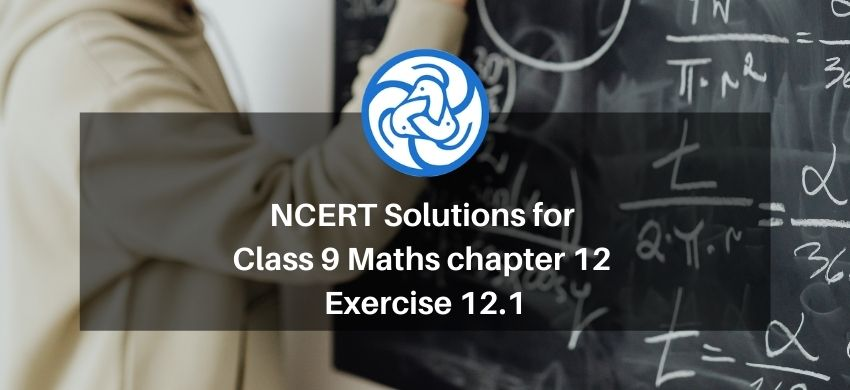Hey, are you a class 9 Student and Looking for Ways to Download NCERT Solutions for Class 9 Maths chapter 12 Exercise 12.1? If Yes then you are at the right place.

Here we have listed Class 9 maths chapter 12 exercise 12.1 solutions in PDF that is prepared by Kota’s top IITian’s Faculties by keeping Simplicity in mind.

If you want to score high in your class 9 Maths Exam then it is very important for you to have a good knowledge of all the important topics, so to learn and practice those topics you can use eSaral NCERT Solutions.

So, without wasting more time Let’s start.

### Download The PDF of NCERT Solutions for Class 9 Maths chapter 12 Exercise 12.1 "Heron’s Formula"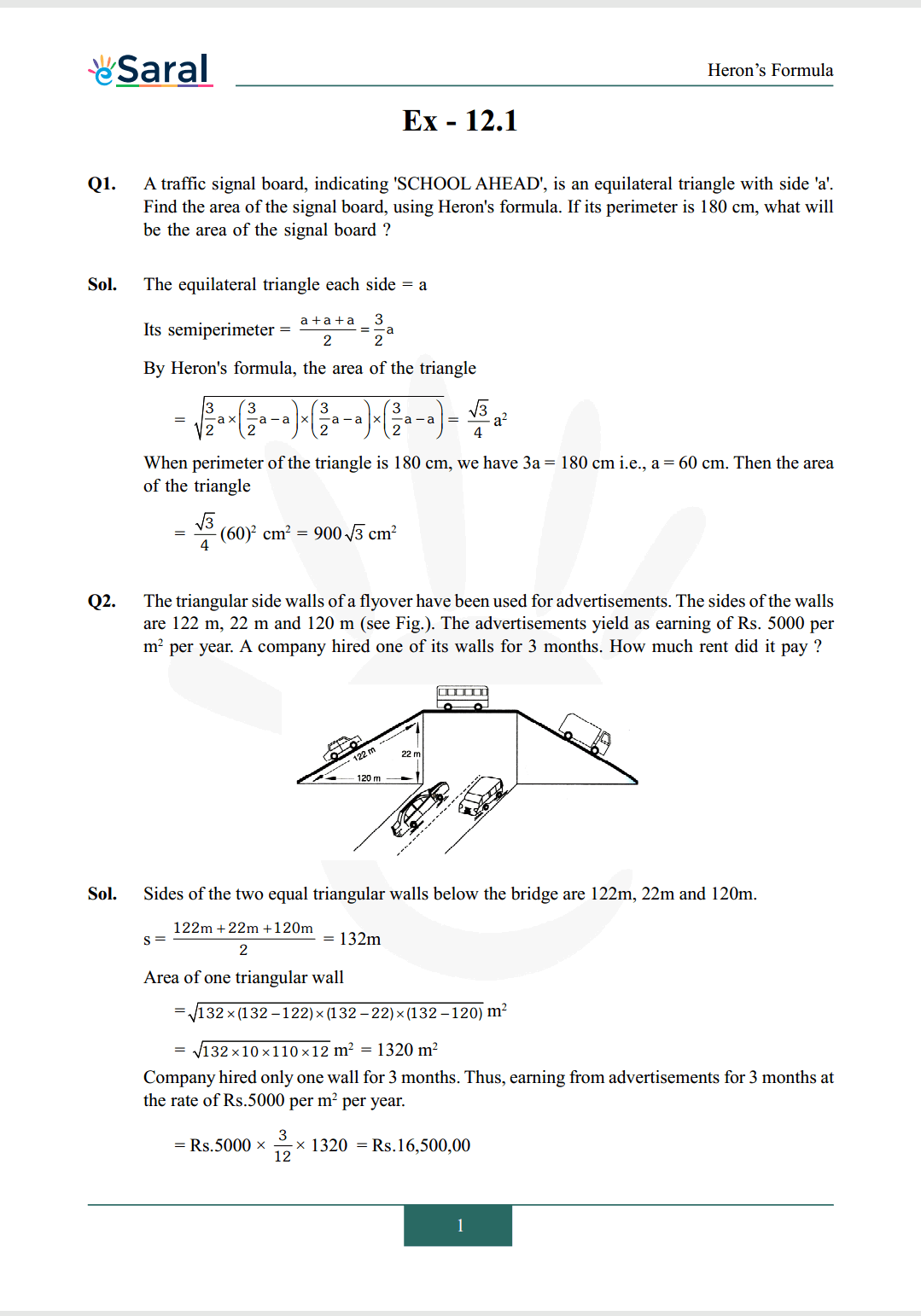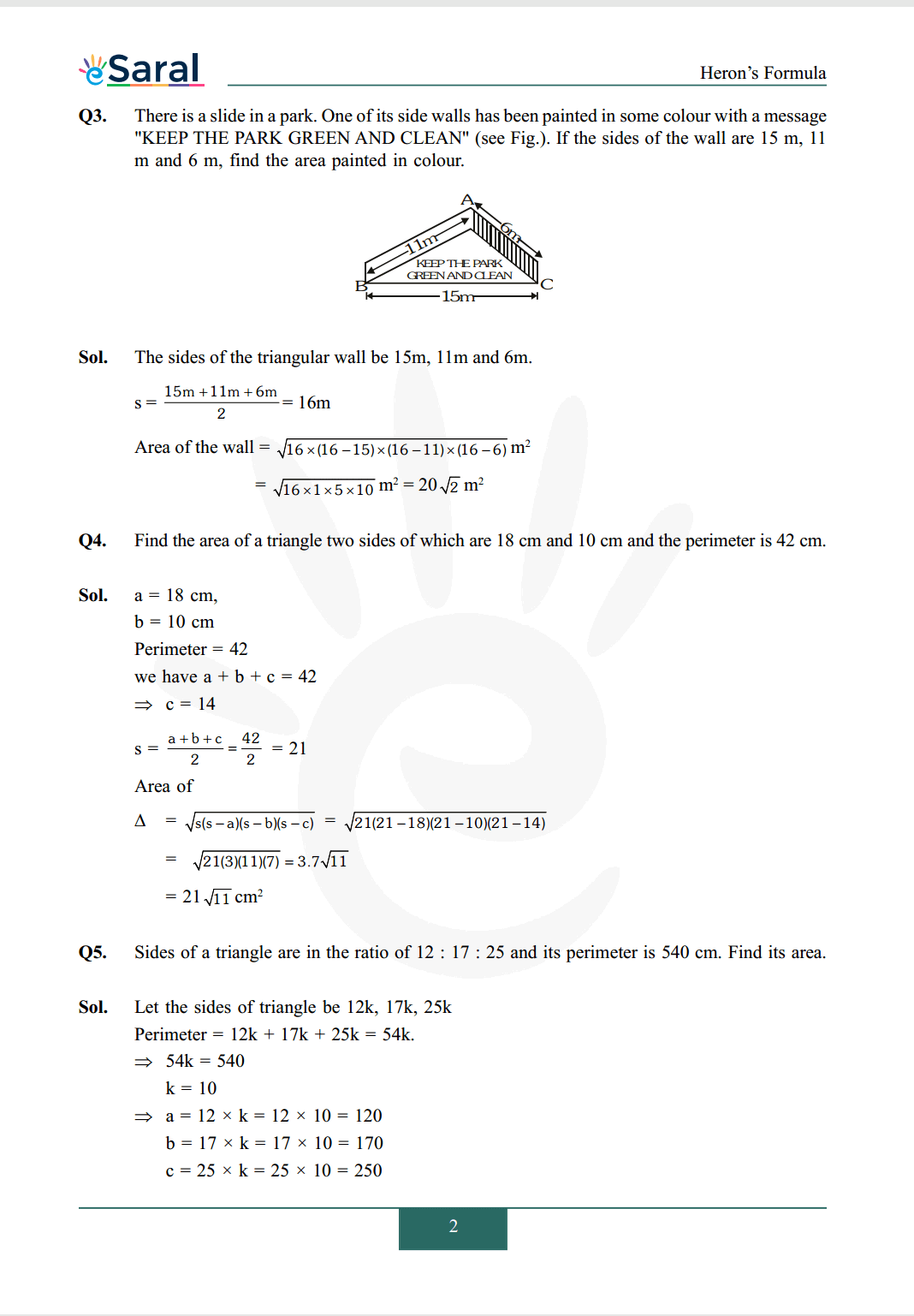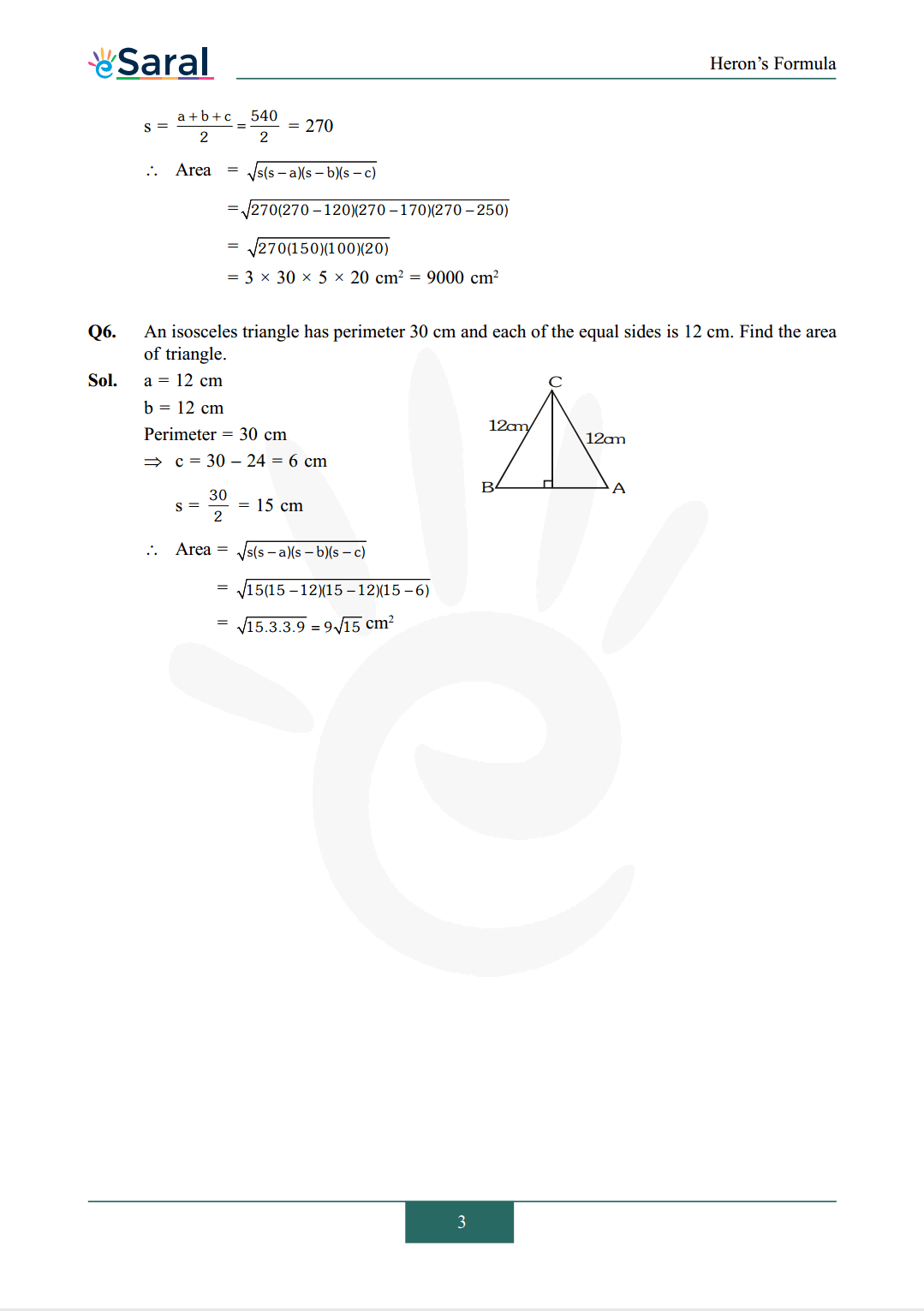#### All Questions of Chapter 12 Exercise 12.1

Once you complete the chapter 12 then you can revise Ex. 12.1 by solving following questions

Q1. A traffic signal board, indicating 'SCHOOL AHEAD', is an equilateral triangle with side 'a'. Find the area of the signal board, using Heron's formula. If its perimeter is $180 \mathrm{~cm}$, what will be the area of the signal board ?

Q2. The triangular side walls of a flyover have been used for advertisements. The sides of the walls are $122 \mathrm{~m}, 22 \mathrm{~m}$ and $120 \mathrm{~m}$ (see Fig.). The advertisements yield as earning of Rs. 5000 per $\mathrm{m}^{2}$ per year. A company hired one of its walls for 3 months. How much rent did it pay ?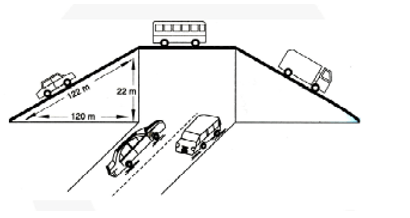Q3. There is a slide in a park. One of its side walls has been painted in some colour with a message "KEEP THE PARK GREEN AND CLEAN" (see Fig.). If the sides of the wall are $15 \mathrm{~m}, 11$ $\mathrm{m}$ and $6 \mathrm{~m}$, find the area painted in colour.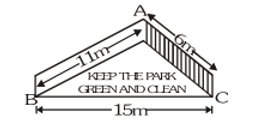Q4. Find the area of a triangle two sides of which are 18 cm and 10 cm and the perimeter is 42 cm.

Q5. Sides of a triangle are in the ratio of 12: 17: 25 and its perimeter is 540 cm. Find its area.

Q6. An isosceles triangle has perimeter 30 cm and each of the equal sides is 12 cm. Find the area of triangle.